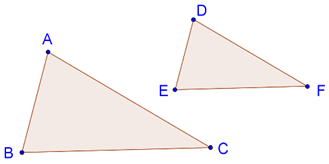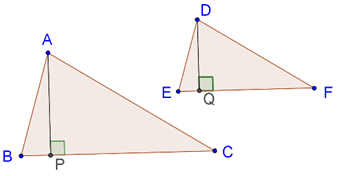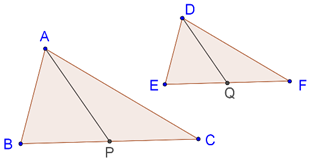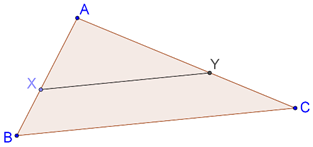# Areas of Similar Triangles

Go back to  'Similar-Triangles'

Two congruent triangles have the same area. What about two similar triangles? What is the relation between their areas? Intuitively, this should be easy to answer. Since two similar triangles are uniformly scaled versions of each other, their bases and heights should be in the same ratio. Therefore, the ratio of their areas should be the square of the ratio of any their sides. Let us prove this rigorously.

Theorem: The ratio of the areas of two similar triangles is equal to the square of the ratio of any pair of their corresponding sides.

Consider the following figure, which shows two similar triangles, $$\Delta ABC$$ and $$\Delta DEF$$:Our theorem tells us that

$\frac{{ar(\Delta ABC)}}{{ar(\Delta DEF)}} = \frac{{A{B^2}}}{{D{E^2}}} = \frac{{B{C^2}}}{{E{F^2}}} = \frac{{A{C^2}}}{{D{F^2}}}$

Proof: Draw the altitudes AP and DQ, as shown below:Note that $$\Delta ABP$$ and $$\Delta DEQ$$ are equi-angular (how?), and hence similar. Thus,

$\frac{{AP}}{{DQ}} = \frac{{AB}}{{DE}}$

This further implies that

$\frac{{AP}}{{DQ}} = \frac{{BC}}{{EF}}$

Thus,

\begin{align}&\frac{ar(\Delta ABC)}{ar(\Delta DEF)}=\frac{\frac12\times BC\times AP}{\frac12\times EF\times DQ}=\left(\frac{BC}{EF}\right)\times\left(\frac{AP}{DQ}\right)\\&\;\;\;\;\;\;\;\;\;\;\;\,\;\;\;\;\;\;\;\;\;\;\;\;\;\;\;\;\;\;\;\;\;\;\;\;\;\;\;\;\;\;\;\;\;\;\;\;\;=\left(\frac{BC}{EF}\right)^2\end{align}

Similarly, we can show that

$\frac{{ar(\Delta ABC)}}{{ar(\Delta DEF)}} = {\left( {\frac{{AB}}{{DE}}} \right)^2} = {\left( {\frac{{AC}}{{DF}}} \right)^2}$

Example 1: Consider two similar triangles, $$\Delta ABC$$ and $$\Delta DEF$$, as shown below:AP and DQ are medians in the two triangles. Show that

$\frac{{ar\Delta (ABC)}}{{A{P^2}}} = \frac{{ar\Delta (DEF)}}{{D{Q^2}}}$

Solution: Since the two triangles are similar, we must also have $$\Delta ABP$$ ~ $$\Delta DEQ$$. We have already proved this earlier, but you may pause for a moment and prove this again. Since these triangles are similar, their areas will be in the ratio of the squares of any pair of corresponding sides. Thus:

\begin{align}& \frac{{ar(\Delta ABC)}}{{ar(\Delta DEF)}} = \frac{{A{P^2}}}{{D{Q^2}}}\\& \Rightarrow \frac{{ar(\Delta ABC)}}{{A{P^2}}} = \frac{{ar(\Delta DEF)}}{{D{Q^2}}} \end{align}

Example 2: Consider the following figure:It is given that XY || AC and divides the triangle into two parts of equal areas. Find the ratio AX:XB.

Solution: Since XY || AC, $$\Delta BXY$$ must be similar to $$\Delta BAC$$. Thus,

\begin{align}& \frac{{ar(\Delta ABC)}}{{ar\left( {\Delta AXY} \right)}} = 2 = \frac{{A{B^2}}}{{A{X^2}}}\\ &\Rightarrow \frac{{AB}}{{AX}} = \sqrt 2 \\& \Rightarrow \frac{{AB}}{{AX}} - 1 = \sqrt 2 - 1\\ &\Rightarrow \frac{{XB}}{{AX}} = \sqrt 2 - 1\\ &\Rightarrow \frac{{AX}}{{XB}} = \frac{1}{{\sqrt 2 - 1}} \end{align}

Learn math from the experts and clarify doubts instantly

• Instant doubt clearing (live one on one)
• Learn from India’s best math teachers
• Completely personalized curriculum